# Special Functions¶

There are a lot of useful special function in physics. Some of them provides physics understanding of the problem, some of them helps us writing down a solution quickly.

Among them, Gamma functions, Legendre polynomials, Bessel functions, spherical harmonics, modified bessel functions, spherical bessel functions, and elliptical functions are the most used ones.

## Gamma Functions¶

Gamma function satisfies the following relatioin,

$\Gamma(z+1) = z\Gamma(z) .$

For some cases, it can also be written as

$\Gamma(n) = \int_0^\infty dt t^{n-1} e^{-t} .$

One can prove that

$\Gamma(z)\Gamma(1-z) = \frac{\pi}{\sin(\pi z)} .$

## Legendre Polynomials¶

Legendre polynomials are solutions to Legendre equation, which is

$\left(\frac{d}{dx}\left[(1-x^2)\frac{d}{dx}\right] + n(n+1)\right) P_n(x) = 0.$

Legendre polynomials has many different representations.

Integral

$P_n(z) = \frac{1}{2\pi i} \oint (1 - 2 t z + t^2)^{1/2} t^{-n-1} dt.$

Rodrigues representation

$P_n(z) = \frac{1}{2^l l!} \frac{d^l}{d x^l} (x^2 - 1)^l .$

It’s generation function is

$\frac{1}{\sqrt{1 + \eta^2 - 2 \eta x }} = \sum_{k=0}^\infty \eta^k P_k(x) .$

Properties

Orthogonality

$\int_{-1}^1 P_m(x) P_n(x) dx = \frac{2}{2n + 1}\delta_{mn} .$

They all have value 1 at $$z=1$$.

The parity is alternating.

Examples

$\begin{split}P_0(x) & = 1 \\ P_1(x) & = x \\ P_2(x) & = \frac{1}{2}(3x^2-1).\end{split}$

Through these, we can solve out

$\begin{split}x &= P_1(x) \\ x^2 &= \frac{1}{3}(P_0(x) + 2 P_2(x) ).\end{split}$

Notice that they have physics meanings although it’s better to understand it together with spherical harmonics.

## Associated Legendre Polynomials¶

The associated Legendre equation is

$\left(\frac{d}{dx}\left[(1-x^2)\frac{d}{dx}\right] + n(n+1) - \frac{m^2}{1-x^2} \right) P_n(x) = 0.$

The solution to this equation is Associated Legendre polynomial, which can be represented by

$P_n^{\nu}(x) = (-1)^m(1-x^2)^{m/2} \frac{d^m}{dx^m} P_l(x) .$

## Bessel Functions¶

Bessel functions are solutions to Bessel equation,

$\left( x \frac{d}{dx} x \frac{d}{dx} + x^2 - \nu^2 \right) J_{\nu} (x) = 0.$

They all satisfy these recurrence relations,

$\begin{split}Z_{n+1} + Z_{n-1} &= \frac{2n}{x} Z_n \\ Z_{n-1} - Z_{n+1} & = 2 \frac{d}{dx}Z_n .\end{split}$

### Bessel Function of the first kind¶

Use notation $$J_n(x)$$ for the first kind.

Generating function is

$e^{\frac{z}{2}\left(t-\frac{1}{t}\right)} = \sum_{n=-\infty}{infty} t^n J_n(z) .$

Integral representation

$J_n(z) = \frac{1}{2\pi} \int_{-\pi}^{\pi} e^{i(n\tau - x \sin \tau)} d\tau .$

It also has a summation representation,

$J_\alpha(z) = \sum_{m=0}^\infty \frac{(-1)^m}{m!\Gamma(m+\alpha +1)} \left( \frac{x}{2} \right)^{2m+\alpha} .$

At large $$\vert x \vert$$ limits, we have

$\begin{split}\lim_{\vert x\vert \to \infty} J_l(x) &= \frac{\sin(z-l\frac{\pi}{2})}{x} \\ \lim_{\vert x\vert \to \infty} J_l'(x) &= \frac{\cos(z-l\frac{\pi}{2})}{x} .\end{split}$

By playing with the recurrence relation,

$\begin{split}2J_n' &= J_{n-1} - J_{n+1} \\ 2n J_n & = J_{n+1} + J_{n-1},\end{split}$

we can get two more useful relations,

$\begin{split}\frac{d}{dz} (z^n J_n) & = z^n J_{n-1} \\ \frac{d}{dz} (z^{-n} J_{n}) & = - z^{-n} J_{n+1} .\end{split}$

They are very useful when integrating by part.

### Graphics and Properties¶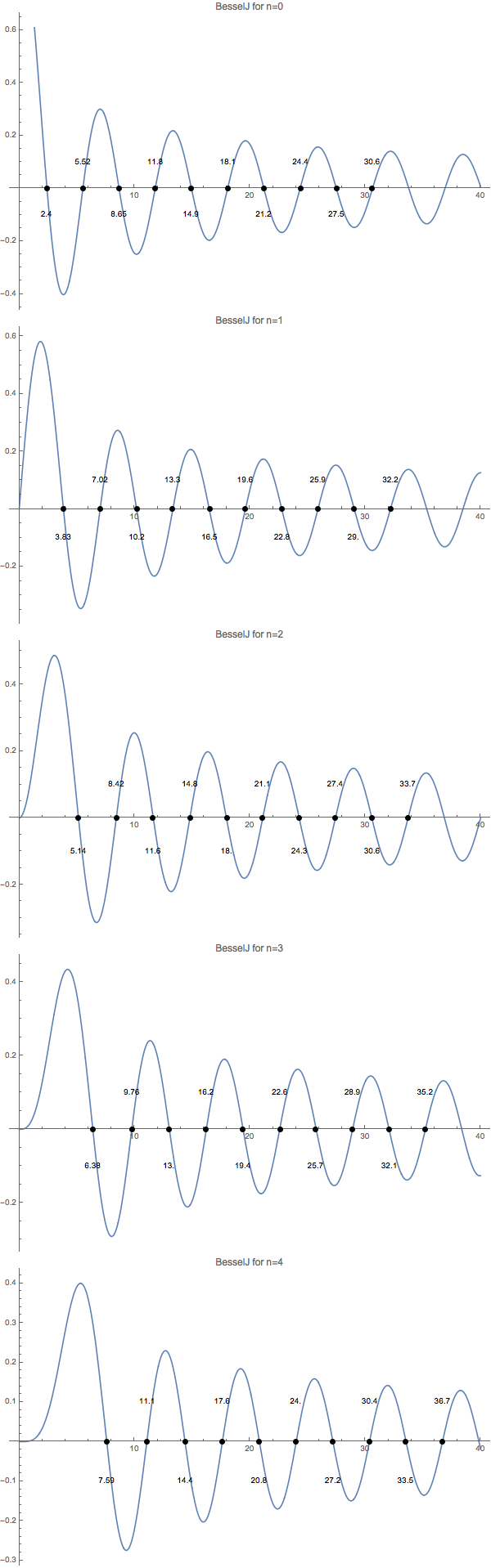Fig. 4 The first 10 zeros of Bessel functions from order 0 to 4.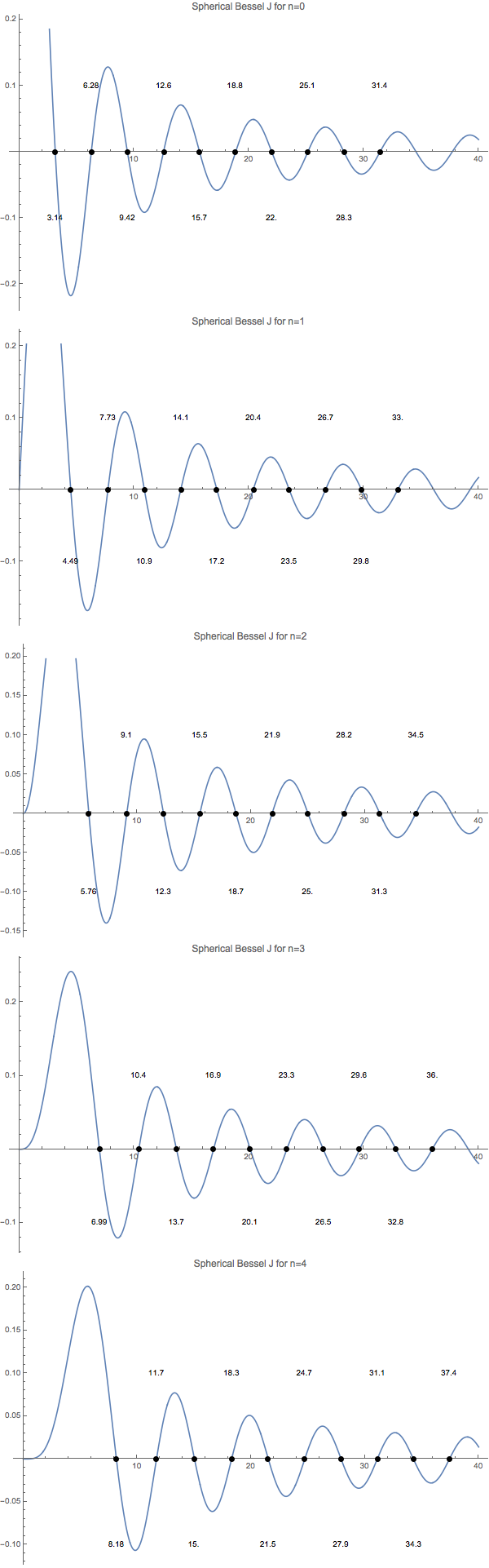Fig. 5 The first 10 zeros of spherical Bessel functions from order 0 to 4.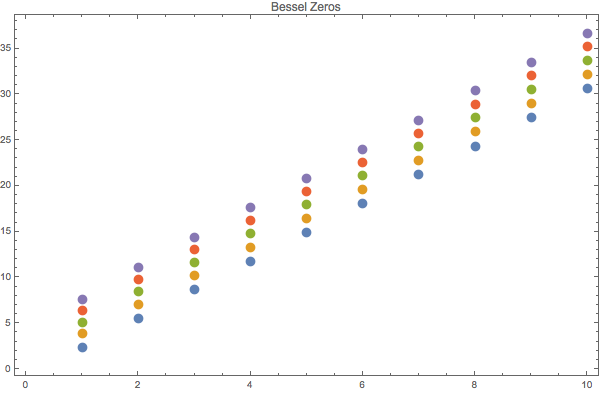Fig. 6 Bessel function zeros in a list plot. Horizontal axis is nth zero point, while vertical axis is the value.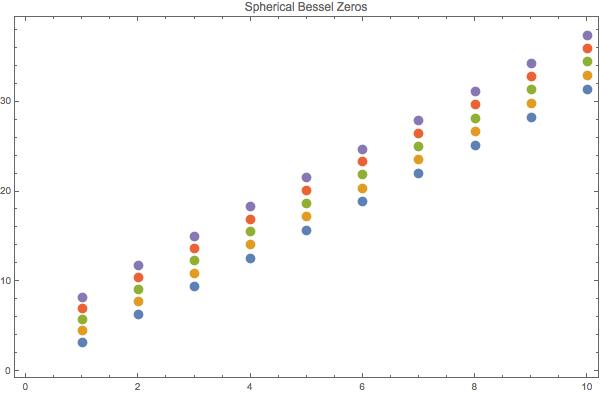Fig. 7 Spherical Bessel function zeros.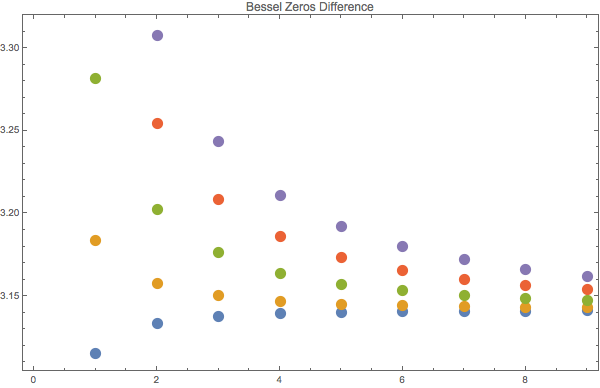Fig. 8 The difference between zeros of Bessel functions. They are almost the same, which a around Pi.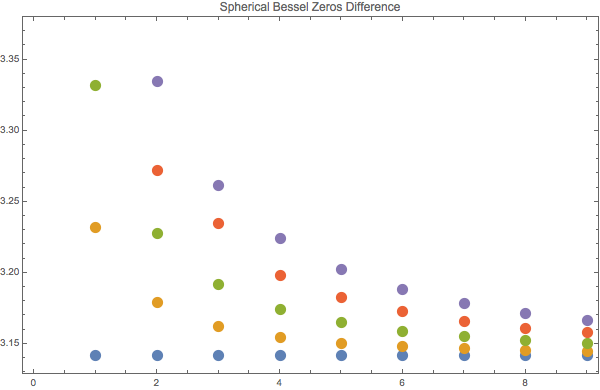Fig. 9 Spherical Bessel function zeros differences.